Test: Quantitative Techniques- 10

# Test: Quantitative Techniques- 10

Test Description

## 20 Questions MCQ Test IBPS PO Prelims- Study Material, Online Tests, Previous Year | Test: Quantitative Techniques- 10

Test: Quantitative Techniques- 10 for Banking Exams 2023 is part of IBPS PO Prelims- Study Material, Online Tests, Previous Year preparation. The Test: Quantitative Techniques- 10 questions and answers have been prepared according to the Banking Exams exam syllabus.The Test: Quantitative Techniques- 10 MCQs are made for Banking Exams 2023 Exam. Find important definitions, questions, notes, meanings, examples, exercises, MCQs and online tests for Test: Quantitative Techniques- 10 below.
Solutions of Test: Quantitative Techniques- 10 questions in English are available as part of our IBPS PO Prelims- Study Material, Online Tests, Previous Year for Banking Exams & Test: Quantitative Techniques- 10 solutions in Hindi for IBPS PO Prelims- Study Material, Online Tests, Previous Year course. Download more important topics, notes, lectures and mock test series for Banking Exams Exam by signing up for free. Attempt Test: Quantitative Techniques- 10 | 20 questions in 30 minutes | Mock test for Banking Exams preparation | Free important questions MCQ to study IBPS PO Prelims- Study Material, Online Tests, Previous Year for Banking Exams Exam | Download free PDF with solutions
 1 Crore+ students have signed up on EduRev. Have you?
Test: Quantitative Techniques- 10 - Question 1

### Study the graph carefully and answer the questions PRODUCTION OF SUGAR (IN TONES) OF THREE DIFFERENT STATES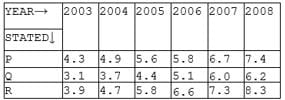Q. What is the approximate percentage increase in production of sugar in state Q from 2006 to 2007?

Detailed Solution for Test: Quantitative Techniques- 10 - Question 1

Required increase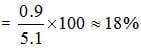Test: Quantitative Techniques- 10 - Question 2

### Study the graph carefully and answer the questions PRODUCTION OF SUGAR (IN TONES) OF THREE DIFFERENT STATES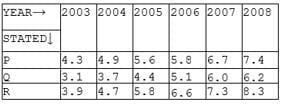Q. What is the average production of sugar of all 3 states in the year 2003 to that 2004?

Detailed Solution for Test: Quantitative Techniques- 10 - Question 2

Required average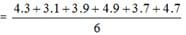= 4.1 tonnes

Test: Quantitative Techniques- 10 - Question 3

### Study the graph carefully and answer the questions PRODUCTION OF SUGAR (IN TONES) OF THREE DIFFERENT STATESQ. What is the ratio of total production of sugar of all three states in the year 2006 to that of 2007?

Detailed Solution for Test: Quantitative Techniques- 10 - Question 3

Required Ratio
= (5.8 + 5.1 + 6.6) : (6.7 + 6.0 + 7.3)
= 17.5 : 20
= 7:8

Test: Quantitative Techniques- 10 - Question 4

Study the graph carefully and answer the questions
PRODUCTION OF SUGAR (IN TONES) OF THREE DIFFERENT STATESQ. What is the average production of state R for all years together?

Detailed Solution for Test: Quantitative Techniques- 10 - Question 4

Required average production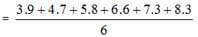= 6.1 tonnes

Test: Quantitative Techniques- 10 - Question 5

Study the graph carefully and answer the questions
PRODUCTION OF SUGAR (IN TONES) OF THREE DIFFERENT STATES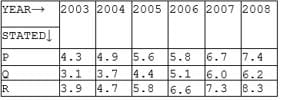Q. What is difference between the total production of all the three states together in 2008 to that in 2005?

Detailed Solution for Test: Quantitative Techniques- 10 - Question 5

Required difference
= (7.4 + 6.2 + 8.3) – (5.6 + 4.4 + 5.8)
= 21.9 – 15.8
= 6.1 tonnes

Test: Quantitative Techniques- 10 - Question 6

√8-√4-√2 = ?

Detailed Solution for Test: Quantitative Techniques- 10 - Question 6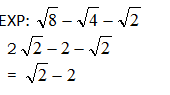Test: Quantitative Techniques- 10 - Question 7

4/7 x 2/3x ?=1008

Detailed Solution for Test: Quantitative Techniques- 10 - Question 7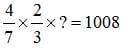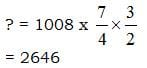Test: Quantitative Techniques- 10 - Question 8

What least number should be added to 5931 to make it exactly divisible by 94?

Detailed Solution for Test: Quantitative Techniques- 10 - Question 8

The correct option is B.
to find the least number that has to be added, find the remainder and subtract this from the divisor.
Here 5931/94; remainder as 9
Subtracting 94-9 = 85.

Test: Quantitative Techniques- 10 - Question 9

If a point (x, y) in a oxy plane is equidistant from (-1, 1) and (4, 3) then?

Detailed Solution for Test: Quantitative Techniques- 10 - Question 9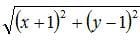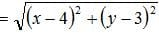x2 + 1 + 2x + y2 + 1 - 2y = x2 + 16 - 8x + y2 + 9 - 6y
10x + 4y = 23

Test: Quantitative Techniques- 10 - Question 10

In how many different ways the letters of the word GYANM be arranged such that Y and M are being on either side?

Detailed Solution for Test: Quantitative Techniques- 10 - Question 10

= 2! X 3!
= 12

Test: Quantitative Techniques- 10 - Question 11

-224-314x (-9) = ?

Detailed Solution for Test: Quantitative Techniques- 10 - Question 11

-224 – 315 x (-9) = ?
-224 + 2826
= 2602

Test: Quantitative Techniques- 10 - Question 12

Find the probability of getting exactly one hand in tossing of two coins?

Detailed Solution for Test: Quantitative Techniques- 10 - Question 12

Required probability =1/4=0.25

Test: Quantitative Techniques- 10 - Question 13

D die is rolled twice, what is the probability that the sum of the numbers on the two faces is 5?

Detailed Solution for Test: Quantitative Techniques- 10 - Question 13

No.Total of possible outcome of outcomes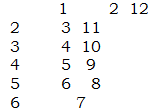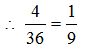Test: Quantitative Techniques- 10 - Question 14

A man standing on a railway platform 100m long, observes that a train crossed the platform in 25 seconds but himself in 15 seconds. Find the length of the train and it’s speed?

Detailed Solution for Test: Quantitative Techniques- 10 - Question 14

PL = 100m.  TL = ? (25 – 15) = 10 sec
Speed of Train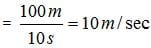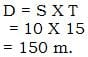Test: Quantitative Techniques- 10 - Question 15

If 30% of a class consists of girls and there are 21 boys, in the class, then how many girls are there in the class?

Detailed Solution for Test: Quantitative Techniques- 10 - Question 15

∴ 70% of class is Boys.
= 70% cl → 21 Boys
Class = 30 students.
∴ Girls = (30 – 21)
= 9.

Test: Quantitative Techniques- 10 - Question 16

Is 377 is a prime number?

Detailed Solution for Test: Quantitative Techniques- 10 - Question 16

Is 377 is a prime number ?
Yes this number is divisible by 13.
∴ This number is not a prime number.
means composite number.

Test: Quantitative Techniques- 10 - Question 17

0, 1, 5, 14, 30, 55, (?)

Detailed Solution for Test: Quantitative Techniques- 10 - Question 17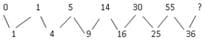So ? = 91

Test: Quantitative Techniques- 10 - Question 18

(?)2  ÷ 4 + √1521 = 1063

Detailed Solution for Test: Quantitative Techniques- 10 - Question 18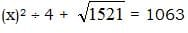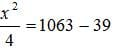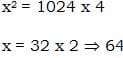Test: Quantitative Techniques- 10 - Question 19

Find the sum of numbers 6 + 7 + 9 + ………… + 20

Detailed Solution for Test: Quantitative Techniques- 10 - Question 19

Find the sum of numbers.
6 + 7 + 9 + ……… + 20.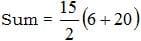= 15 x 13
= 195

Test: Quantitative Techniques- 10 - Question 20

How much time it will take for a boy to run around a square park of side 35m with speed of 9km/hr?

Detailed Solution for Test: Quantitative Techniques- 10 - Question 20

Square park of side 35 m.
Distance = 35 x 4
= 140m.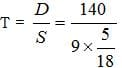⇒ 28 x 2
⇒ 56 sec.

## IBPS PO Prelims- Study Material, Online Tests, Previous Year

173 videos|127 docs|149 tests
 Use Code STAYHOME200 and get INR 200 additional OFF Use Coupon Code
Information about Test: Quantitative Techniques- 10 Page
In this test you can find the Exam questions for Test: Quantitative Techniques- 10 solved & explained in the simplest way possible. Besides giving Questions and answers for Test: Quantitative Techniques- 10, EduRev gives you an ample number of Online tests for practice

## IBPS PO Prelims- Study Material, Online Tests, Previous Year

173 videos|127 docs|149 tests